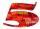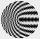# Unknown number

Unknown number is divisible by exactly three different primes. When we compare these primes in ascending order, the following applies:
• Difference first and second prime number is half the difference between the third and second prime numbers.
• The product of the difference the second with the first primes
and difference the third and the second prime number is a multiple of 17.

Determine the smallest number that has all the above properties.

Result

n =  2014

#### Solution:

2014= 2 × 19 × 53
$primes = array(2,3,5,7,11,13,17,19,23,29,31,37,41,43,47,53,59,61,67); for($a=0;$a<count($primes); $a++) { for($b=$a+1;$b<count($primes);$b++)
{
for($c=$b+1;$c<count($primes); $c++) {$p1 = $primes[$a];
$p2 =$primes[$b];$p3 = $primes[$c];

if($p2-$p1 == 0.5*($p3-$p2) &&  mod(($p2-$p1)*($p3-$p2),17)==0)
{
$rv[$p1*$p2*$p3] = "$p1$p2 $p3"; } } } } ksort($rv);
print_r(\$rv);

$2014= 2 \cdot 19 \cdot 53$

Leave us a comment of this math problem and its solution (i.e. if it is still somewhat unclear...):Be the first to comment!## Next similar math problems:

1. DivisorsThe sum of all divisors unknown odd number is 2112. Determine sum of all divisors of number which is twice of unknown numbers.
2. Theorem proveWe want to prove the sentence: If the natural number n is divisible by six, then n is divisible by three. From what assumption we started?
3. CombinationsHow many different combinations of two-digit number divisible by 4 arises from the digits 3, 5 and 7?
4. Amazing numberAn amazing number is name for such even number, the decomposition product of prime numbers has exactly three not necessarily different factors and the sum of all its divisors is equal to twice that number. Find all amazing numbers.
5. NumbersWrite smallest three-digit number, which in division 5 and 7 gives the rest 2.
6. Chebyshev formulaTo estimate the number of primes less than x is used Chebyshev formula: ? Estimate the number of primes less than 30300537.
7. Sum of two primesChristian Goldbach, a mathematician, found out that every even number greater than 2 can be expressed as a sum of two prime numbers. Write or express 2018 as a sum of two prime numbers.
8. BlocksThere are 9 interactive basic building blocks of an organization. How many two-blocks combinations are there?
9. Three unknownsSolve the system of linear equations with three unknowns: A + B + C = 14 B - A - C = 4 2A - B + C = 0
10. PIN - codesHow many five-digit PIN - code can we create using the even numbers?
11. CandiesIn the box are 12 candies that look the same. Three of them are filled with nougat, five by nuts, four by cream. At least how many candies must Ivan choose to satisfy itself that the selection of two with the same filling? ?
12. CardsSuppose that are three cards in the hats. One is red on both sides, one of which is black on both sides, and a third one side red and the second black. We are pulled out of a hat randomly one card and we see that one side of it is red. What is the probabi
13. Elimination methodSolve system of linear equations by elimination method: 5/2x + 3/5y= 4/15 1/2x + 2/5y= 2/15
14. Today in schoolThere are 9 girls and 11 boys in the class today. What is the probability that Suzan will go to the board today?
15. AP - simpleDetermine the first nine elements of sequence if a10 = -1 and d = 4
16. LegsCancer has 5 pairs of legs. The insect has 6 legs. 60 animals have a total of 500 legs. How much more are cancers than insects?
17. LineIt is true that the lines that do not intersect are parallel?